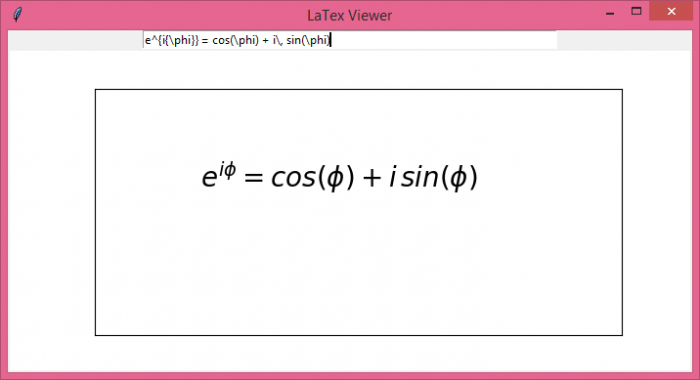# How to display LaTex in real time in a text box in Tkinter?

TkinterPythonGUI-Programming

Python Matplotlib library is useful in applications where we need to visualize the data points and draw graphs and plots to analyze the data. Let us suppose that we want to create a tkinter application where we can process LaTex syntax.

LaTex syntax is used for preparing scientific documentation such as, formulae, scientific notations, mathematical characters, and punctuations. To prepare the application, we are required to use matplotlib and TkAgg (backend API for Matplotlib in Tkinter) modules. The following steps are used to structure the application functions and widgets,

• Import the required libraries such as Matplotlib, Tkinter, Ttk (for styling the widgets), TkAgg, etc.
• Add a Frame and define a label and an Entry widget in it.
• Define the size of the Figure using the figure() method in Matplotlib. This figure can be used to plot the syntax in canvas.
• Now, create a Canvas widget where we will define our figure using TkAgg.
• Define a function to get the content of the Entry widget and convert the text in a figure using the predefined function in Matplotlib, i.e., text().
• Display the output on the canvas after binding the Return or Click event with the function.

## Example

# Import required libraries
from tkinter import *
from tkinter import ttk
import matplotlib
import matplotlib.pyplot as plt
from matplotlib.backends.backend_tkagg import FigureCanvasTkAgg

# Use TkAgg in the backend of tkinter application
matplotlib.use('TkAgg')

# Create an instance of tkinter frame
win = Tk()

# Set the size of the window
win.geometry("700x350")

# Set the title of the window
win.title("LaTex Viewer")

# Define a function to get the figure output
def graph(text):
# Get the Entry Input
tmptext = entry.get()
tmptext = "$"+tmptext+"$"
# Clear any previous Syntax from the figure
wx.clear()
wx.text(0.2, 0.6, tmptext, fontsize = 20)
canvas.draw()
# Create a Frame object
frame = Frame(win)
frame.pack()
# Create an Entry widget
var = StringVar()
entry = Entry(frame, width=70, textvariable=var)
entry.pack()

# Add a label widget in the frame
label = Label(frame)
label.pack()

# Define the figure size and plot the figure
fig = matplotlib.figure.Figure(figsize=(7, 4), dpi=100)
canvas = FigureCanvasTkAgg(fig, master=label)
canvas.get_tk_widget().pack(side=TOP, fill=BOTH, expand=1)
canvas._tkcanvas.pack(side=TOP, fill=BOTH, expand=1)

# Set the visibility of the Canvas figure
wx.get_xaxis().set_visible(False)
wx.get_yaxis().set_visible(False)

win.bind('<Return>', graph)
win.mainloop()

## Output

Executing the above code will display a window with an Entry widget and a figure plot. Now, type some scientific expression to show the resultant output in LaTex format.